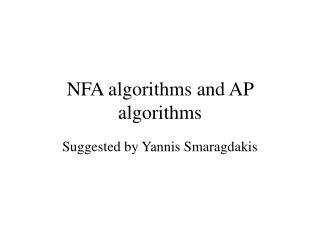DownloadDownload PresentationNFA algorithms and AP algorithms

# NFA algorithms and AP algorithms

Download Presentation## NFA algorithms and AP algorithms

- - - - - - - - - - - - - - - - - - - - - - - - - - - E N D - - - - - - - - - - - - - - - - - - - - - - - - - - -
##### Presentation Transcript

1. NFA algorithms and AP algorithms Suggested by Yannis Smaragdakis

2. Integrated view of algorithms 1 and 2: for path existence • Both are similar to the intersection of two NDFAs • Algorithm 1 (Traversal Graph Algorithm): NDFA for strategy graph and NDFA for class graph: results in NDFA for traversal graph. • Algorithm 2: NDFA for traversal graph and NDFA for object graph: results in NDFA which tells us whether there is a non-empty traversal

3. Traversal Graph AlgorithmExample 1 s1 q1 q2 q3 t1 any any A B C D A=B D. B=C. C=D. D=. s2 q6 q7 t2 D A C D from A via C to D s1,s2 q1,q6 q2,q6 q3,q7 t1,t2 A B C D Traversal Graph

4. Traversal Graph AlgorithmExample 2 A s1 q1 q2 q3 t1 any any B C D A A=B D. B=C. C=D. D=[A]. s2 q6 q7 t2 D t1,q7 A A C D from A via C to D D s1,s2 q1,q6 q2,q6 q3,q7 t1,t2 A B C D Traversal Graph, after some simplification can be further simplified by merging (t1,t2) and (t1,q7)

5. Intersection of NDFA is similar to traversal graph construction q4 b a q2 q3 q1 a b a b q2q4 q1q3 q1q4 Intersection of two DFAs

6. Recall: Intersection of NDFAs • An NDFA is a 5-tuple: M=(S,A,d,p0,F), S finite set of states, A is input alphabet, d is a state transition function which maps Ax(S union epsilon) to the set of subsets of S, p0 is the initial state, and F is the set of final states.

7. Recall: Intersection of NDFAs • M1=(S1,A,d1,p0,F1) and M2=(S2,A,d2,q0,F2). • The NDFA for M1 intersect M2 is I=(S1xS2,A,d,(p0,q0),F1xF2), where for a in A, (p2,q2) in d((p1,q1),a) if and only if p2 in d1(p1,a) and q2 in d2(q1,a).